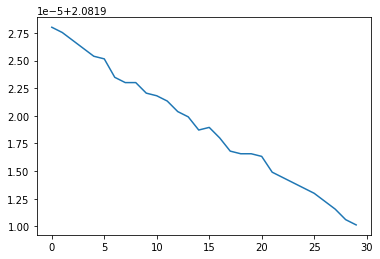# Why does use of mini-batches have such effect on my classifier?

I am learning pytorch and coded a minimal classifier to play with:

``````import torch
import numpy as np
import matplotlib.pyplot as plt

numclasses, count = 8, 200
x = torch.randn(count, 4)
y = torch.randint(0, numclasses, size=[count])

dataset = torch.utils.data.TensorDataset(x, y)

model =  torch.nn.Sequential(
torch.nn.Linear(4, 20),
torch.nn.ReLU(),
torch.nn.Linear(20, numclasses),
torch.nn.Softmax(dim=1))

lossf = torch.nn.CrossEntropyLoss()
optimizer = torch.optim.SGD(model.parameters(), lr=1e-3)

losses = []
for epoch in range(30):
x, y = batch
out = model(x)
loss = lossf(out, y)

loss.backward()
optimizer.step()

losses.append( loss.item() )

plt.plot(losses)
``````

This is what I get with full dataset (batch_size = 200):And this is with mini-batch (batch_size = 20):Any idea why this happens?

Could this ever happen in real problems with real data?

The larger the batch size, the less noise the parameter updates will include.
Often this noise is beneficial to reach a better final accuracy, but it might depend on your use case.